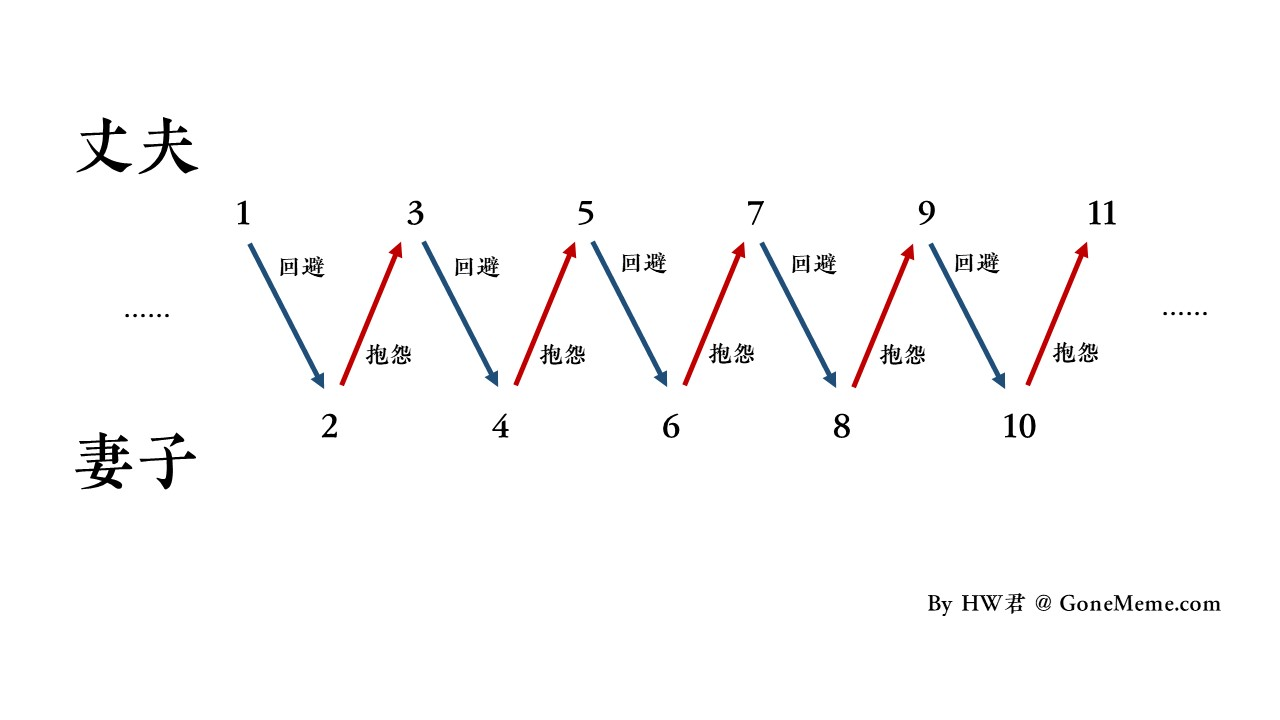文 | HW君

# 1. 无穷的悖论

S = a-a+a-a+a-a+a-a+a-a+a-…

(1)  S = 0

S = (a-a)+(a-a)+(a-a)+(a-a)+…

S = 0+0+0+0+…

S = 0

(2)  S = a

S = a-(a-a)-(a-a)-(a-a)-…

S = a-0-0-0-…

S = a

(3)  S = a/2

S = a-(a-a+a-a+a-a+a-…)

S = a-S

S = a/2

# 2. 无穷事件序列……

（本章节完，敬请期待一下节）

By HW君 @ 2020-11-250 评论